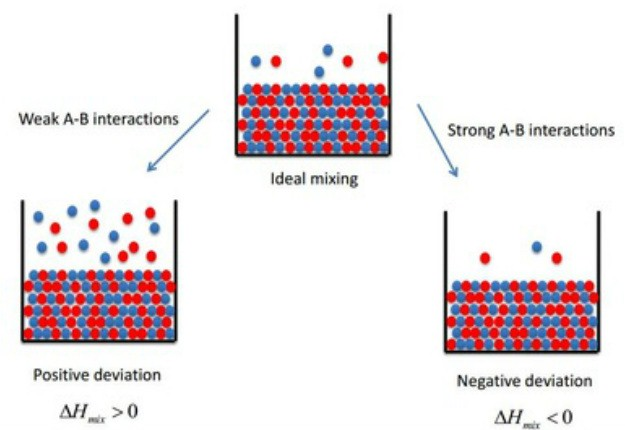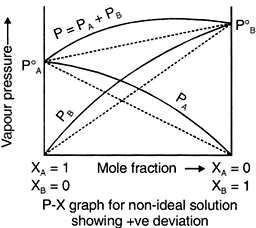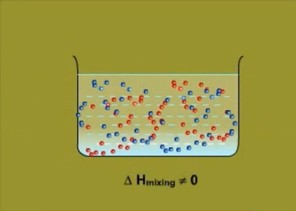×#### Thank you for registering.

One of our academic counsellors will contact you within 1 working day.

Click to Chat

1800-1023-196

+91-120-4616500

CART 0

• 0

MY CART (5)

Use Coupon: CART20 and get 20% off on all online Study Material

ITEM
DETAILS
MRP
DISCOUNT
FINAL PRICE
Total Price: Rs.

There are no items in this cart.
Continue Shopping```Ideal and Non-Ideal Solutions

Raoult’s Law

Ideal Solutions

Non- Ideal Solutions

Positive Deviation from Raoult’s Law

Negative Deviation from Raoult’s Law

Azeotrope

Maximum Boiling Azeotrope

Minimum Boiling Azeotrope

Raoult’s Law

In 1986, a French Chemist named Francois Marte Raoult proposed a quantitative relation between partial pressure and mole fraction of volatile liquids. The law states that mole fraction of the solute component is directly proportional to its partial pressure.Image 1: Types of Solutions

On the basis of Raoult’s Law, liquid-liquid solutions are classified into two types of solutions, they are:

Ideal Solutions

Non-ideal Solutions

Ideal Solutions

The solutions which obey Raoult’s Law at every range of concentration and at all temperatures are called Ideal Solutions. We can obtain ideal solutions by mixing two ideal components that is, solute and a solvent having similar molecular size and structure. For Example, consider two liquids A and B, and mix them. The formed solution will experience several intermolecular forces of attractions inside it, which will be:

A – A intermolecular forces of attraction

B – B intermolecular forces of attraction

A – B intermolecular forces of attraction

The solution is said to be an ideal solution, only when the intermolecular forces of attraction between A – A, B – B and A – B are nearly equal.Image 2: Molecular structure is same in case of ideal solutions

Ideal Solutions generally have characteristics as follows:

They follow Raoult’s Law, which means partial pressure of components A and B in a solution will be PA = PA0 xA and PB = PB0 xB  where PA0 and PB0 are respective vapour pressure in pure form and xA and xB are respective mole fractions of components A and B

The enthalpy of mixing of two components should be zero, that is, Δmix H = 0. This signifies that no heat is released or absorbed during mixing of two pure components to form ideal solution

The volume of mixing of two components should be zero that is, Δmix V = 0. This means that total volume of solution is equal to the sum of the volume of solute and solution. Adding further, it also signifies that there is no occurrence of contraction or expansion of volume while mixing of two components

The solute-solute interaction and solvent-solvent interaction is nearly equal to solute-solvent interaction

Note: Perfectly ideal solutions are rare in nature, only some solutions show some ideal behavior.

Examples of Ideal Solutions

n-hexane and n-heptane

Bromoethane and Chloroethane

Benzene and Toluene

CCl4 and SiCl4

Chlorobenzene and Bromobenzene

Ethyl Bromide and Ethyl Iodide

n-Butyl Chloride and n-Butyl Bromide

Non-Ideal Solutions

The solutions which don’t obey Raoult’s law at every range of concentration and at all temperatures are called Non-Ideal Solutions. Non-ideal solutions deviate from ideal solutions and are also known as Non-Ideal Solutions.Image 3: Graph between vapour pressuring and mole fractionImage 4: The enthalpy of mixing is non-zero in non-ideal solution as some heat is released or absorbed in the process.

Non-ideal solutions depict characteristics as follows:

The solute-solute and solvent-solvent interaction is different from that of solute-solvent interaction

The enthalpy of mixing that is, Δmix H ≠ 0, which means that heat might have released if enthalpy of mixing is negative  (Δmix H < 0) or the heat might have observed if enthalpy of mixing is positive (Δmix H > 0)

The volume of mixing that is,  Δmix V ≠ 0, which depicts that there will be some expansion or contraction in dissolution of liquids

Non-ideal solutions are of two types:

Non-ideal solutions showing positive deviation from Raoult’s Law

Non-ideal solutions showing negative deviation from Raoult’s Law

Positive Deviation from Raoult’s Law

Positive Deviation from Raoult’s Law occurs when the vapour pressure of component is greater than what is expected in Raoult’s Law. For Example, consider two components A and B to form non-ideal solutions. Let the vapour pressure, pure vapour pressure and mole fraction of component A be PA , PA0 and xA respectively and that of component B be PB , PB0 and xB respectively. These liquids will show positive deviation when Raoult’s Law when:

PA > PA0 xA and PB > P0B xB, as the total vapour pressure (PA0 xA + P0B xB) is greater than what it should be according to Raoult’s Law.

The solute-solvent forces of attraction is weaker than solute-solute and solvent-solvent interaction that is, A – B < A – A or B – B

The enthalpy of mixing is positive that is, Δmix H > 0 because the heat absorbed to form new molecular interaction is less than the heat released on breaking of original molecular interaction

The volume of mixing is positive that is, Δmix V > 0 as the volume expands on dissolution of components A and B

Examples: Following are examples of solutions showing positive deviation from Raoult’s Law

Acetone and Carbon disulphide

Acetone and Benzene

Carbon Tetrachloride and Toluene or Chloroform

Methyl Alcohol and Water

Acetone and Ethanol

Ethanol and Water

Negative Deviation from Raoult’s Law

Negative Deviation occurs when the total vapour pressure is less than what it should be according to Raoult’s Law. Considering the same A and B components to form a non-ideal solution, it will show negative deviation from Raoult’s Law only when:

PA < PA0 xA and PB < P0B xB as the total vapour pressure (PA0 xA + P0B xB) is less than what it should be with respect to Raoult’s Law

The solute-solvent interaction is stronger than solute-solute and solvent-solvent interaction that is, A – B > A – A or B – B

The enthalpy of mixing is negative that is, Δmix H < 0 because more heat is released when new molecular interactions are formed

The volume of mixing is negative that is,  Δmix V < 0 as the volume decreases on dissolution of components A and B

Examples: Following are examples of solutions showing negative deviation from Raoult’s Law

Chloroform and Benzene

Chloroform and Diether

Acetone and Aniline

Nitric Acid ( HNO3) and water

Acetic Acid and pyridine

Hydrochloric Acid ( HCl) and waterImage 5: Graph between vapour pressure and mole fraction

Azeotropes

Azeotropes are defined as a mixture of two liquids which has a constant composition in liquid and vapour phase at all temperatures. Azeotropes can’t be separated by fractional distillation, as the composition of vapour phase remains same after boiling. Because of uniform composition azeotropes are also known as Constant Boiling Mixtures.

There are two types of Azeotropes:

Maximum Boiling Azeotrope

Minimum Boiling Azeotrope

Maximum Boiling Azeotrope

Maximum Boiling Azeotrope is formed when we mix two non-ideal solutions at some specific composition, showing large negative deviation from Raoult’s law.

Examples:

Nitric Acid (HNO3) (68%) and water (32%) form maximum boiling azeotrope at boiling temperature of 393.5 K

Hydrochloric Acid (HCl) (20.24%) and water form maximum boiling azeotrope at boiling temperature of 373 K

Minimum Boiling Azeotrope

Minimum Boiling Azeotrope is formed when we mix two non-ideal solutions at some specific composition, which shows large positive deviation from Raoult’s Law.

Example:

Ethanol ( 95.5 %) and water ( 4.5 %) form minimum boiling azeotrope at boiling temperature of 351.5 K

Watch this Video for more reference

Ideal and Non-Ideal Solutions
```### Course Features

• 731 Video Lectures
• Revision Notes
• Previous Year Papers
• Mind Map
• Study Planner
• NCERT Solutions
• Discussion Forum
• Test paper with Video Solution